• python怎么求最大公约数和最小公倍数 一、求最大公约数 用辗转相除法求最大公约数的算法如下： 两个正整数a和b（a>b），它们的最大公约数等于a除以b的余数c和b之间的最大公约数。比如10和25，25除以10商2余5，那么10...
• 算法设计与分析 实 验 报 告 书 实验名称 算法设计与分析之实验一 两个数的最大公约数 学 号 2012210890 姓 名 王朔 评语 成绩 指导教师 批阅时间 年 月 日 算法分析与设计实验报告 - 1 - 一 实验目的和要求 1 ...
• 主要介绍了PHP编程求最大公约数与最小公倍数的方法,涉及php数学计算的相关运算技巧,需要的朋友可以参考下
• C++ 实现求最大公约数和最小公倍数 最大公约数 辗转相除法: int maxDivisor(int a, int b) { int c = b; while (a%b != 0) { c = a%b; a = b; b = c; } return c; } 辗转相减法： int maxDivisor(int a,...
• 课程的随堂作业，C语言的，用dev就能运行，萌新代码，勿喷，仅仅帮助不想写作业的朋友方便一下，反正老师也不会仔细检查的
• 今天整理了一下用递归法求最大公约数(gcd)和最小公倍数(lcm)。主要的工作是求最大公约数。数学上可以用辗转法求最大公约数
• 主要介绍了java求最大公约数与最小公倍数的方法,涉及java数值运算的相关操作技巧,并附带分析了eclipse环境下设置运行输入参数的相关操作技巧,需要的朋友可以参考下
•编程语言
• 有关c++求最大公约数的代码，用的是辗转相除法，很简单的算法过程，主要是求最大公约数
• C++求最大公约数的四种方法思路，供大家参考，具体内容如下 将最近学的求最大公约数的四种方法总结如下： 第一种：穷举法之一 解释：拿其中一个数出来，用一个临时变量（tem）保存，每次都把那两个数除以这个临时...
• ## 求最大公约数的4种算法（C++）

万次阅读 多人点赞 2019-03-16 00:37:03
求最大公约数的4种算法（C++） 一、实验目的 1.计算两个正整数的最大公约数和最小公倍数，并进行程序的调式与测试。 2.理解四种不同的求最大公约数的方法，学习其思维模式。 3.了解算法的概念。对问题的分析时，...
求最大公约数的4种算法（C++）
一、实验目的 1.计算两个正整数的最大公约数和最小公倍数，并进行程序的调式与测试。 2.理解四种不同的求最大公约数的方法，学习其思维模式。 3.了解算法的概念。对问题的分析时，进行合适的算法设计。 二、算法设计 1.题目分析 （1）首先输入一对正整数，对两个数进行判断，若不是正整数，则给出提示并重新输入，直至两个数均为正整数为止。 （2）将两个数传入计算最大公约数的函数和计算最小公倍数的函数中，求解输出。 （3）验证各种算法的效率，在求解函数之前设置一个计时器开始运行，在函数执行完毕后，结束计时，计算出两个时间节点的差，即可得出每个函数执行一定次数的时间。规定统一的次数，将每种算法所用时间进行对比即可。 2.算法设计 1.辗转相除法，又称欧几里得算法。用于计算两个正整数a，b的最大公约数和最小公倍数，其依赖于gcd(a,b) = a (b=0)和gcd(b,a mod b) (b!=0). 2．穷举法，也叫枚举法，求最大公约数时从两者中较小的数开始，由大到小列举，直到找到第一个公约数为止。 3.更相减损法，若两个正整数都为偶数，则用2约简，直到不能约简为止。然后用大数减小数，将差与较小的数比较，再以大数减小数，直到减数和差相等为止。 4. Stein算法，两个数均为偶数时有公约数2，且公约数一定为偶数。一个奇数一个偶数时，因为其奇偶性的不同，所以其最大公约数一定为奇数。当两个数均为奇数时，其最大公约数一定是奇数。 三、业务流程图设计 欧几里得算法：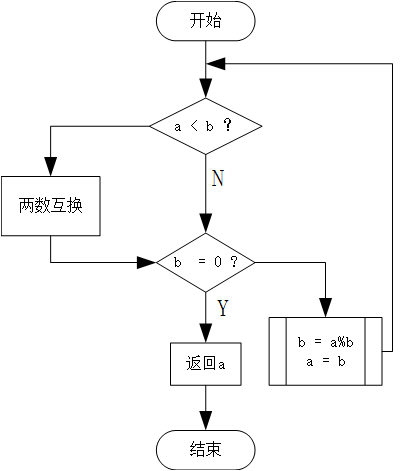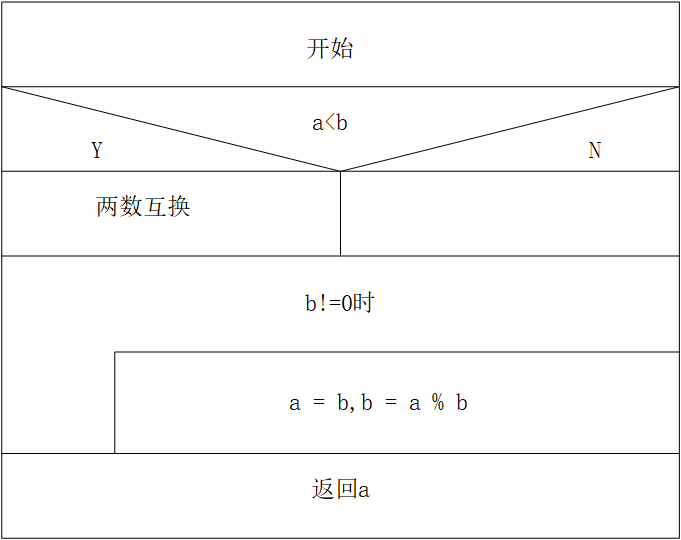穷举法：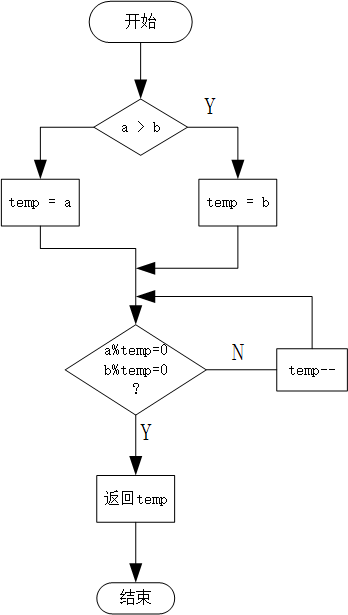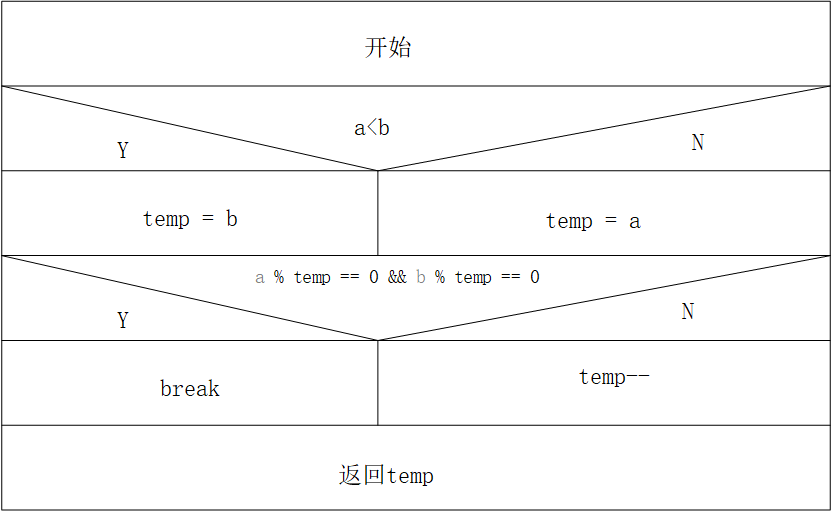更相减损法：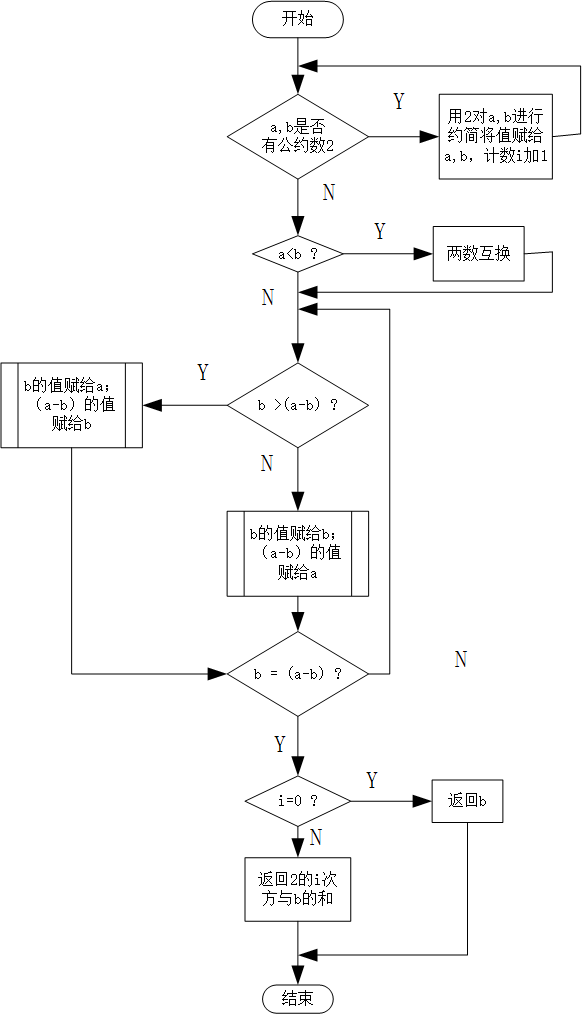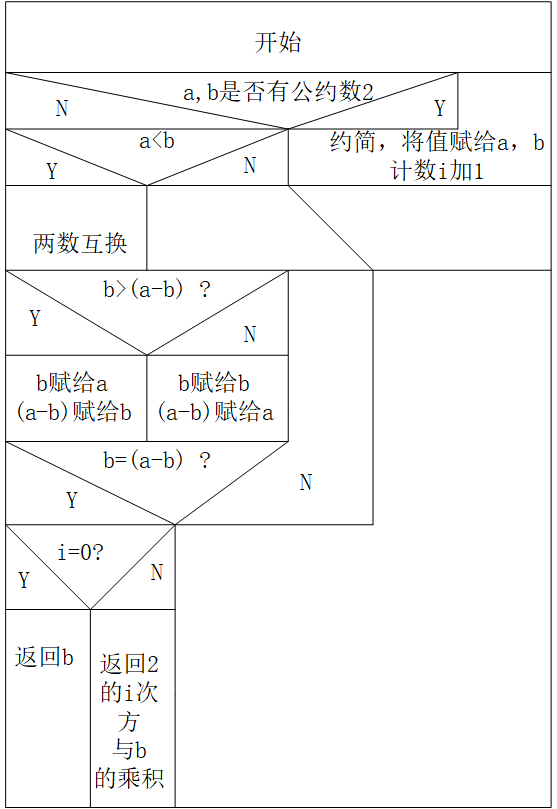Stein算法：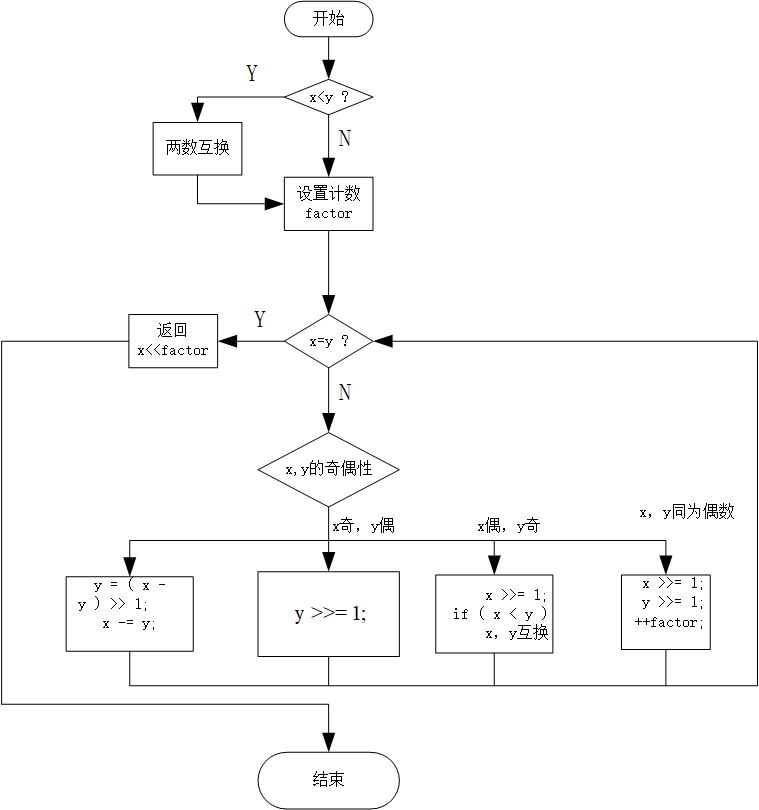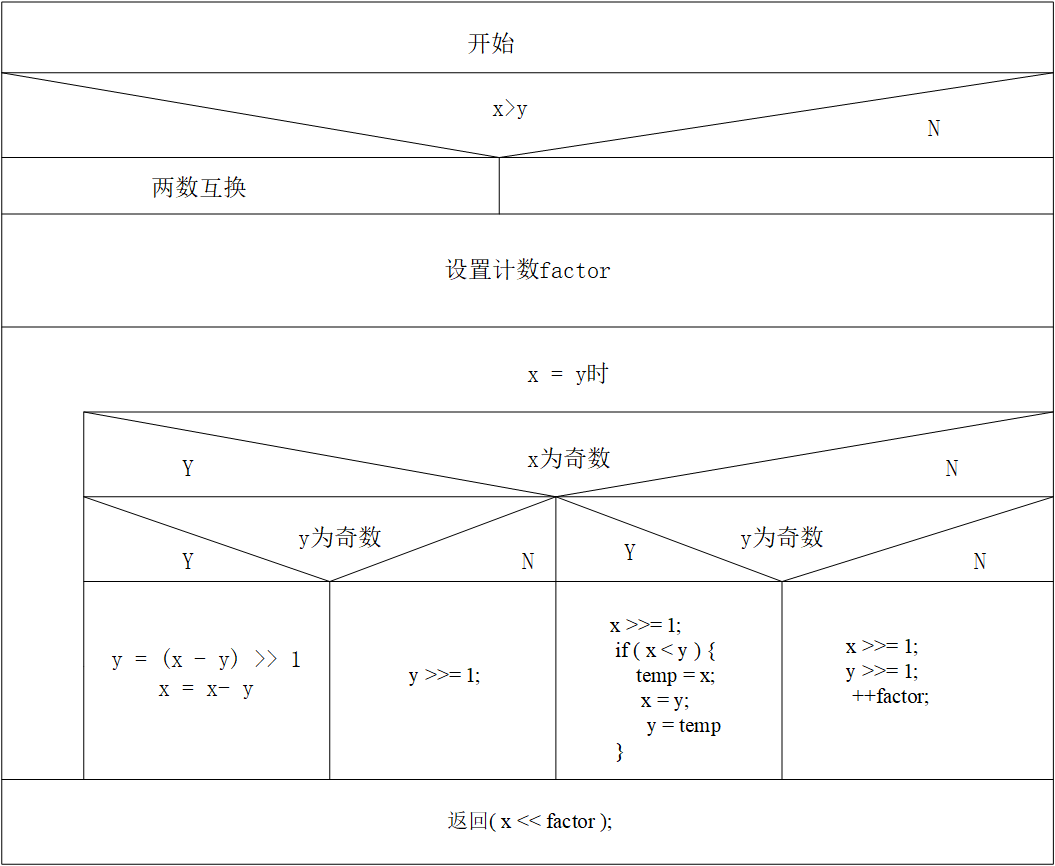四、主要代码（2.0.0版）
/*********************************************************************************
*FileName:  Test1.cpp
*Author:  Qixiang.Su
*e-mail:  617992304@qq.com
*Version:  2.0.0
*Date:  2019.3.10
*Description: 求两个正整数的最大公约数和最小公倍数
*             理解各种算法求解过程
*History:
1.Date:    2019.3.10
Author:  Elf.苏洛曦
Modification:   Create  project
2.Date:    2019.3.12
Author: Elf.苏洛曦
Modification:   1.0.0版
3.Date:    2019.3.14
Author:  Elf.苏洛曦
Modification:   2.0.0版
**********************************************************************************/
#include<iostream>
#include<time.h>
using namespace std;

int t1, t2, s, w;

//辗转相除法求最大公约数函数
int divisor(int a, int b) {
int temp;

//比较两个数的大小，值大的数为a，值小的数为b
if (a < b) {
temp = a;
a = b;
b = temp;
}

//求余
while (b != 0) {
temp = a % b;
a = b;
b = temp;
}
return a;
}

//求最小公倍数
int multiple(int a, int b) {
int divisor(int a, int b);
int temp;
temp = divisor(a, b);
return(a * b / temp);
}

//函数递归调用求最大公约数
int gcd(int a, int b) {
if (a % b == 0) {
return b;
}
else {
return gcd(b, a % b);
}
}

//穷举法求最大公约数
int divisor1(int a, int b) {
int temp;
temp = (a > b) ? b : a;   //求较小值
while (temp > 0) {
if (a % temp == 0 && b % temp == 0) {
break;
}
else {
temp--;
}
}
return (temp);
}

//穷举法求最小公倍数
int multiple1(int a, int b) {
int p, q, temp;
p = (a > b) ? a : b;  //求两数中的最大值
q = (a > b) ? b : a;  //求两数中的最小值
temp = p;
while (1) {
if (p % q == 0) {
break;
}
else {
p += temp;
}
}
return (p);
}

//更相减损法求最大公约数
int gcd1(int a, int b) {
int i = 0, temp, x = 0;
while (a % 2 == 0 && b % 2 == 0) {    //m,n有公约数2时
a /= 2;
b /= 2;
i += 1;
}
//a,b的值互换
if (a < b) {
temp = a;
a = b;
b = temp;
}
while (x) {
x = a - b;
a = (b > x) ? b : x;
b = (b < x) ? b : x;
if (b == (a - b)) {    //差和减数相等
break;
}
}
if (i == 0) {
return b;
}
else {
return (int)pow(2, i)*b;
}
}

//输入正整数
int setNumber() {
int a;
cout << "请输入正整数：" << endl;
cin >> a;
if (a <= 0) {
cout << "您输入的数值不符合规范（要求：正整数)" << endl;
setNumber();
}
else {
return a;
}
}

//Stein算法函数非递归调用求最大公约数
int Stein(unsigned int x, unsigned int y) {
int factor = 0;   //计数器
int temp;

//大数赋给x，小数赋给y
if (x < y) {
temp = x;
x = y;
y = temp;
}
if (0 == y) {
return 0;
}
while (x != y) {
if (x & 0x1) {
if (y & 0x1) {   //x,y都为奇数
y = (x - y) >> 1;
x -= y;
}
else {    // x为奇数，y为偶数
y >>= 1;
}
}
else {
if (y & 0x1) {   // x为偶数，y为奇数
x >>= 1;
if (x < y) {
temp = x;
x = y;
y = temp;
}
}
else {   //x,y均为偶数
x >>= 1;
y >>= 1;
++factor;
}
}
}
return (x << factor);
}

//Stein算法函数递归调用求最大公约数
int gcd2(int u, int v) {
if (u == 0) {
return v;
}
if (v == 0) {
return u;
}
if (~u & 1) {
if (v & 1) {
return gcd2(u >> 1, v);
}
else {
return gcd2(u >> 1, v >> 1) << 1;
}
}
if (~v & 1) {
return gcd2(u, v >> 1);
}
if (u > v) {
return gcd2((u - v) >> 1, v);
}
return gcd2((v - u) >> 1, u);
}

//获得两个正整数的最大公约数和最小公倍数
void getResult() {
s = setNumber();
w = setNumber();

int count;
cout << "请输入你想循环的次数：" << endl;
cin >> count;
clock_t strat = clock();
for (int i = 0; i < count; i++) {
t1 = divisor(s, w);
t2 = multiple(s, w);
}
clock_t end = clock();
cout << "辗转相除法函数嵌套调用所求结果:" << endl;
cout << s << "和" << w << "的最大公约数为：" << t1 << endl;
cout << s << "和" << w << "的最小公倍数为：" << t2 << endl;
cout << "运行" << count << "次所花时间为：" << end - strat << "毫秒"<<endl;
cout << endl;

clock_t strat1 = clock();
for (int i = 0; i < count; i++) {
t1 = gcd(s, w);
t2 = multiple(s, w);
}
clock_t end1 = clock();
cout << "辗转相除法函数递归调用所求结果:" << endl;
cout << s << "和" << w << "的最大公约数为：" << t1 << endl;
cout << s << "和" << w << "的最小公倍数为：" << t2 << endl;
cout << "运行" << count << "次所花时间为：" << end1 - strat1 << "毫秒" << endl;
cout << endl;

clock_t strat2 = clock();
for (int i = 0; i < count; i++) {
t1 = divisor1(s, w);
t2 = multiple1(s, w);
}
clock_t end2 = clock();
cout << "穷举法所求结果:" << endl;
cout << s << "和" << w << "的最大公约数为：" << t1 << endl;
cout << s << "和" << w << "的最小公倍数为：" << t2 << endl;
cout << "运行" << count << "次所花时间为：" << end2 - strat2 << "毫秒" << endl;
cout << endl;

clock_t strat3 = clock();
for (int i = 0; i < count; i++) {
t1 = gcd(s, w);
t2 = multiple(s, w);
}
clock_t end3 = clock();
cout << "更相减损法所求结果:" << endl;
cout << s << "和" << w << "的最大公约数为：" << t1 << endl;
cout << s << "和" << w << "的最小公倍数为：" << t2 << endl;
cout << "运行" << count << "次所花时间为：" << end3 - strat3 << "毫秒" << endl;
cout << endl;

clock_t strat4 = clock();
for (int i = 0; i < count; i++) {
t1 = Stein(s, w);
t2 = multiple(s, w);
}
clock_t end4 = clock();
cout << "Stein算法非递归调用所求结果:" << endl;
cout << s << "和" << w << "的最大公约数为：" << t1 << endl;
cout << s << "和" << w << "的最小公倍数为：" << t2 << endl;
cout << "运行" << count << "次所花时间为：" << end4 - strat4 << "毫秒" << endl;
cout << endl;

clock_t strat5 = clock();
for (int i = 0; i < count; i++) {
t1 = gcd2(s, w);
t2 = multiple(s, w);
}
clock_t end5 = clock();
cout << "Stein算法递归调用所求结果:" << endl;
cout << s << "和" << w << "的最大公约数为：" << t1 << endl;
cout << s << "和" << w << "的最小公倍数为：" << t2 << endl;
cout << "运行" << count << "次所花时间为：" << end5 - strat5 << "毫秒" << endl;
cout << endl;

}

int main() {
getResult();
system("pause");
}

五、调试和测试 1.在1.0.0版本中 一次进行测试，出现了错误，如下图：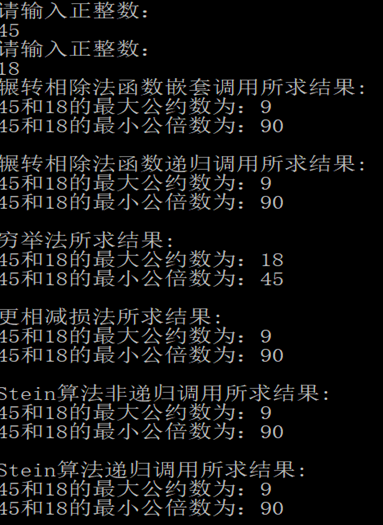在编译时没有错误，在运行过程也无错误，在结果中，穷举法的结果与其他的不同。对穷举法进行单独测试，结果如下：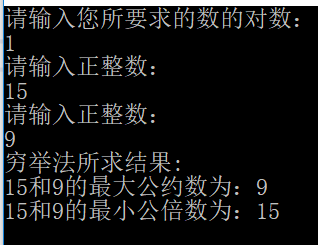与实际不符，结果也是错误的，通过逐语句调试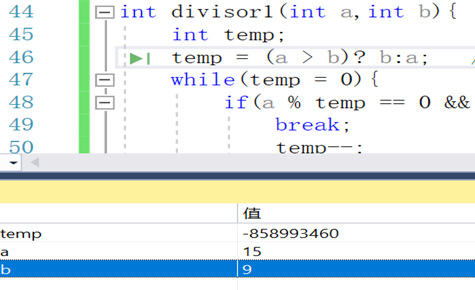发现求最大公约数中temp的值存在异常，通过仔细检查，将while(temp > 0)写成了while(temp = 0)，嵌套出现了层次错误。改正后，运行结果如下：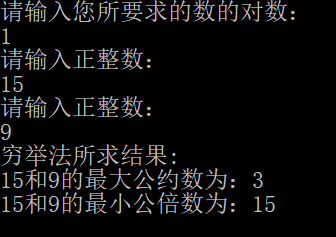最大公约数正确，最小公倍数错误，对求最小公倍数的函数进行逐语句后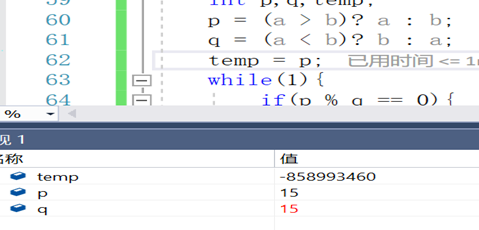p和q的值相同，存在错误，仔细检查后发现将(a>b)写成了(a<b),改正过后，结果均正确，如下图：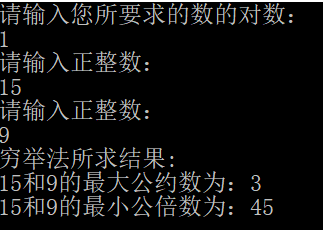2. 2.0.0版本 在2.0.0版本中取消了1.0.0版本中的输入要求数的对数，增加了对一组数据想要循环次数的输入，这样免去了测试时手动输入多组数据的程序。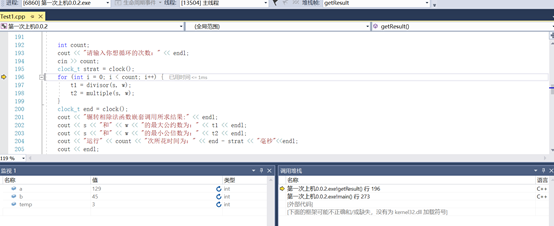通过监视，查看各个变量的变化情况,如下图：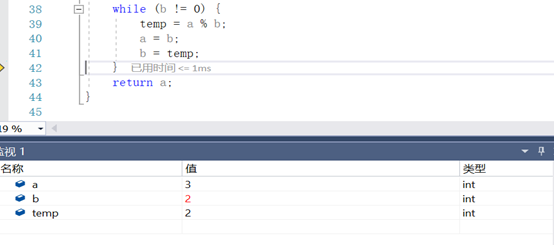（1） 输入两个正整数15和9，执行求解代码100000次，比较各种算法的速度，结果如下图：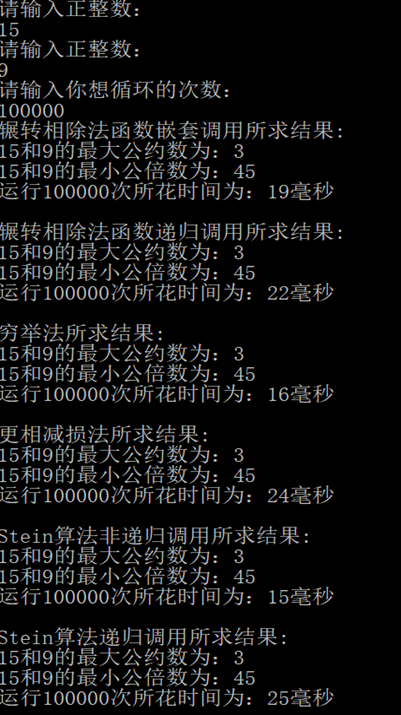从结果可以看出Stein算法非递归比较快，数字较小时，穷举法所用时间也比较短。
（2）输入两个正整数143和345，执行求解代码100000次，比较各种算法的速度，结果如下图：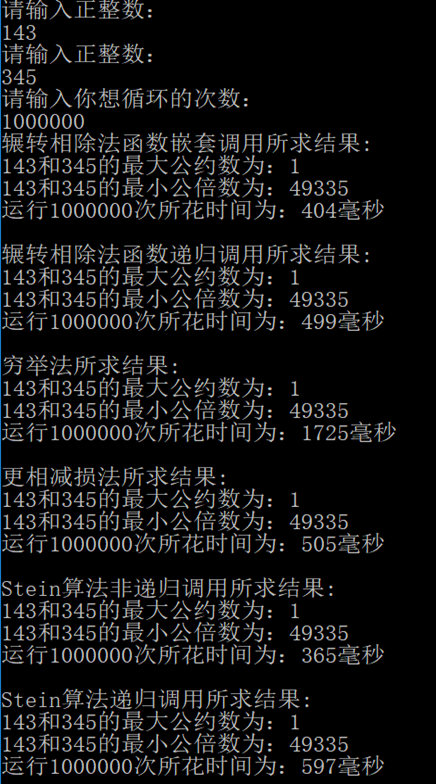在数字较大时且函数循环次数较多之后，Stein算法的执行速度还是很快，而穷举法需要的时间大幅度增长，其他几种算法的时间相差不大。 （3）输入两个正整数12和8，执行求解代码100000次，比较各种算法的速度，结果如下图：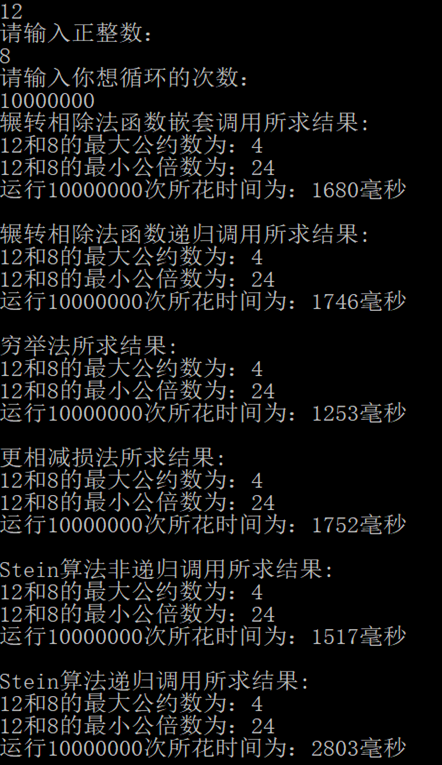数字较小时，穷举法的优势还是很明显，执行速度优于其他几种算法。 同时通过上面的几组测试发现，非递归的速度要优于递归。 六、总结 通过本次实验，学习到了求最大公约数几种常见的算法，对算法也有了一定的认识。也领略到了算法的思维的精妙之处。在上机过程中也出现了一些错误，通过逐步调试也解决了问题。在程序上，还存在一些缺陷，在进行速度的测试时，仅用一组数据的循环次数来判断，这种情况下，就忽略了手动输入数据时数据的复杂性。在输入正整数时仅对数字的正负性进行了判断，而对于其他会出现异常的情况，如在输入数字时误输入字母、符号等，未进行处理。没有通过正则表达式来判断输入，也未将异常进行捕捉和抛出。所以要学习的设计方法还有很多，只有学习到更多的处理方法，才能让自己的程序更具健壮性。
展开全文算法
• C语言编写利用程序递归求最大公约数，递归调用被继承的基类成员函数C语言
• 求最大公约数的代码，利用辗转相处法。Java的基础知识。Java代码
• 包含了：1.辗转相除法函数嵌套流程图2.辗转相除法函数递归流程图3.穷举法最小公倍数流程图4.穷举法求最大公约数流程图5.更相减损术流程图流程图 算法
• 本文实例讲述了Python自定义函数实现两个数最大公约数、最小公倍数。分享给大家供大家参考，具体如下： 1. 最小公倍数的算法: 最小公倍数 = 两个整数的乘积 / 最大公约数 所以我们首先要求出两个整数的最大公...
• 输入两个正整数可以选择不同的算法去计算其最大公约数
• 用欧几里得算法求最大公约数的c++代码，很完整，可以运行
• 分解质因数求最大公约数 输入两个正整数 以空格隔开 即可求得
• 辗转相除法， 又名欧几里德算法(Euclidean algorithm)，是两个正整数之最大公约数的算法。它是已知最古老的算法， 其可追溯至公元前300年前。 它的具体做法是：用较大数除以较小数，再用出现的余数（第一余数）...
辗转相除法
辗转相除法， 又名欧几里德算法(Euclidean algorithm)，是求两个正整数之最大公约数的算法。它是已知最古老的算法， 其可追溯至公元前300年前。
它的具体做法是：用较大数除以较小数，再用出现的余数（第一余数）去除除数，再用出现的余数（第二余数）去除第一余数，如此反复，直到最后余数是0为止。如果是求两个数的最大公约数，那么最后的除数就是这两个数的最大公约数。
另一种求两数的最大公约数的方法是更相减损法。

来源
设两数为a、b(a>b)，求a和b最大公约数(a，b)的步骤如下:用a除以b，得a÷b=q…r1(0≤r1)。若r1=0，则(a，b)=b;若r1≠0，则再用b除以r1，得b÷r1=q…r2 (0≤r2).若r2=0，则(a，b)=r1，若r2≠0，则继续用r1除以r2，如此下去，直到能整除为止。其最后一个为被除数的余数的除数即为(a, b)。  例如:a=25,b=15，a%b=10,b%10=5,10%5=0,最后一个为被除数余数的除数就是5,5就是所求最大公约数。

原理
设两数为a、b(a>b)，用gcd(a,b)表示a，b的最大公约数，r=a (mod b) 为a除以b的余数，k为a除以b的商，即a÷b=kr。辗转相除法即是要证明gcd(a,b)=gcd(b,r)。
第一步:令c=gcd(a,b)，则设a=mc，b=nc
第二步:根据前提可知r =a-kb=mc-knc=(m-kn)c
第三步:根据第二步结果可知c也是r的因数
第四步:可以断定m-kn与n互质(假设m-kn=xd，n=yd (d>1)，则m=kn+xd=kyd+xd=(ky+x)d，则a=mc=(ky+x)cd，b=nc=ycd，则a与b的一个公约数cd>c，故c非a与b的最大公约数，与前面结论矛盾)，因此c也是b与r的最大公约数。
从而可知gcd(b,r)=c，继而gcd(a,b)=gcd(b,r)。
证毕。
以上步骤的操作是建立在刚开始时r≠0的基础之上的。即m与n亦互质。

AC
c++
#include <iostream>
#include <algorithm>
#include <cstdio>
using namespace std;
int gcd(int m,int n){
int r;
r=m%n;
if(r==0)
return n;
return gcd(n,r);
}
int main(){
int m,n;
cout<<"请输入2个整数："<<endl;
cin>>m>>n;
cout<<m<<","<<n<<"最大公约数为："<<gcd(m,n)<<endl;
return 0;
}

展开全文gcd
• 利用素因子分解求最大公约数和最小公倍数 例： 168 和 300 的最大公约数和最小公倍数 解：对 168 和 300 做素因子分解： 168=23×3×7,300=22×3×5168 = 2^{3}\times 3\times 7, 300 = 2^{2}\times 3\times 5168=...
预备知识
定理1：令a，b，c为整数，其中a ≠ 0，则     （i）  如果a | b和a | c，则a | (b + c)；     （ii） 如果a | b，那么对所有整数c都有a | bc；     （iii）如果a | b，b | c，则a | c。
证    下面给出一个 (i) 的直接证明。假定a | b和a | c。则从整除性定义可知，存在整数 s 和 t 满足b = as和c = at。因此

b + c = as + at = a(s + t)

于是，a整除b + c。(ii) 和 (iii) 的证明略。
推论1：如果a，b，c是整数，其中a ≠ 0，使得a | b和a | c，那么当 m 和 n 是整数时有 a | mb + nc。
证    采用直接证明法。由定理1中的 (ii) 可知，当 m 和 n 是整数时有 a | mb 和 a | nc。再由定理1中的 (i) 可得a | mb + nc。
定理2：除数算法(division algorithm)。令 a 为整数，d 为正整数。则存在唯一的整数 q 和 r，满足 0 ≤ r < d，使得a = dq + r。
详细证明请参考《离散数学及其应用》5.2.5节-利用良序性证明
欧几里得算法
我们用gcd(a,b)表示a与b的最大公约数，lcm(a,b)表示a与b的最小公倍数。
1.利用素因子分解求最大公约数和最小公倍数 例：求 168 和 300 的最大公约数和最小公倍数 解：对 168 和 300 做素因子分解：

168

=

2

3

×

3

×

7

,

300

=

2

2

×

3

×

5

168 = 2^{3}\times 3\times 7, 300 = 2^{2}\times 3\times 5

可把它们写成

168

=

2

3

×

3

1

×

5

0

×

7

1

,

300

=

2

2

×

3

1

×

5

2

×

7

0

168 = 2^{3}\times 3^{1}\times5^{0}\times 7^{1}, 300 = 2^{2}\times 3^{1}\times 5^{2}\times7^{0}

于是

g

c

d

(

168

,

300

)

=

2

2

×

3

1

×

5

0

×

7

0

=

12

gcd(168,300) = 2^{2}\times 3^{1}\times5^{0}\times 7^{0} = 12

l

c

m

(

168

,

300

)

=

2

3

×

3

1

×

5

2

×

7

1

=

4200

lcm(168,300) = 2^{3}\times 3^{1}\times5^{2}\times 7^{1} = 4200

2.欧几里得算法又称辗转相除法求最大公约数 直接从整数的素因子分解式计算两个整数的最大公约数效率很低，原因是寻找素因子分解式非常耗时，而欧几里得算法非常高效。 欧几里得算法的基础是下面关于最大公约数和整除算法的结论。
引理1：令a = bq + r，其中a，b，q 和 r均为整数，则 gcd(a,b) = gcd(b,r)
证：如果能证明 a 与 b 的公约数和 b 与 r 的公约数相同，也就证明了 gcd(a,b) = gcd(b,r)，因为这两对整数必定有相同的最大公约数。       因此，假定 d 整除 a 和 b，则可得 d 也整除 a - bq = r (根据推论1)。因此，a 和 b 的任何公约数也是 b 和 r 的公约数。       类似地，假定 d 整除 b 和 r，则可得 d 也整除 bq + r = a。因此，b 和 r 的任何公约数也是 a 和 b 的公约数。       因此，gdc(a,b) = gcd(b,r)。       假定 a 和 b 为正整数，且 a ≥ b。令

r

0

=

a

r_{0}=a

和

r

1

=

b

r_{1}=b

。当连续应用整除算法时，可得

r

0

=

r

1

q

1

+

r

2

0

⩽

r

2

<

r

1

r_{0}= r_{1}q_{1}+ r_{2} \, \, \, \, \, \, \, \, 0 \leqslant r_{2}< r_{1}

r

1

=

r

2

q

2

+

r

3

0

⩽

r

3

<

r

2

r_{1}= r_{2}q_{2}+ r_{3} \, \, \, \, \, \, \, \, 0 \leqslant r_{3}< r_{2}

⋮

\vdots

r

n

−

2

=

r

n

−

1

q

n

−

1

+

r

n

0

⩽

r

n

<

r

n

−

1

r_{n-2}= r_{n-1}q_{n-1}+ r_{n} \, \, \, \, \, \, \, \, 0 \leqslant r_{n}< r_{n-1}

r

n

−

1

=

r

n

q

n

r_{n-1}= r_{n}q_{n} \, \, \, \, \, \, \, \,

最终在这一连续相除序列中会出现余数为0，因为在余数序列

a

=

r

0

>

r

1

>

r

2

>

⋯

⩾

0

a = r_{0}> r_{1}> r_{2}> \cdots \geqslant 0

中至多包含a项，再者从引理1可知

g

d

c

(

a

,

b

)

=

g

c

d

(

r

0

,

r

1

)

=

g

c

d

(

r

1

,

r

2

)

=

⋯

=

gcd

⁡

(

r

n

−

2

,

r

n

−

1

)

gdc(a,b)=gcd(r_{0},r_{1})=gcd(r_{1},r_{2})= \cdots =\gcd(r_{n-2},r_{n-1})

=

g

c

d

(

r

n

−

1

,

r

n

)

=

g

c

d

(

r

n

,

0

)

=

r

n

=gcd(r_{n-1},r_{n})=gcd(r_{n},0)=r_{n}

因此，最大公约数是除法序列中最后一个非零余数。 例：用欧几里得算法寻找 414 和 662 的最大公约数。 解：连续相除得：

662

=

414

⋅

1

+

248

662=414\cdot 1+248

414

=

248

⋅

1

+

166

414=248\cdot 1+166

248

=

166

⋅

1

+

82

248=166\cdot 1+82

166

=

82

⋅

2

+

2

166=82\cdot 2+2

82

=

2

⋅

41

82=2\cdot 41

因此，

g

c

d

(

414

,

662

)

=

2

gcd(414,662)=2

，因为2是最后一个非零余数。
算法和具体实现如下：
算法 欧几里得算法
procedure gcd(a,b:正整数)
x := a
y := b
while y ≠ 0
r := x mod y
x := y
y := r
return x{gcd(a,b)是x}

欧几里得算法实现
int gcd(int a, int b)
{
int x = a;
int y = b;
int r;				// 余数
while (y != 0) {
r = x % y;
x = y;
y = r;
}
return x;
}

3.求最小公倍数 除了运用1中的质因数分解法外，可以用公式法求两个数的最小公倍数。由于两个数的乘积等于这两个数的最大公约数与最小公倍数的积。即

a

×

b

=

g

c

d

(

a

,

b

)

×

l

c

m

(

a

,

b

)

a \times b = gcd(a,b)\times lcm(a,b)

。所以，求两个数的最小公倍数，就可以先求出它们的最大公约数，然后用上述公式求出它们的最小公倍数。
展开全文• 两个正整数m和n的最大公约数。 输入样例1： 6 8 输出样例1： 2 //递归求最大公约数 #include<stdio.h> int f(int a,int b) { //比大小,确定被除数和除数 //a为被除数，b为除数 if(b>a) { int ...

求两个正整数m和n的最大公约数。 输入样例1： 6 8 输出样例1： 2

//递归求最大公约数
#include<stdio.h>

int f(int a,int b)
{
//比大小,确定被除数和除数
//a为被除数，b为除数
if(b>a)
{
int temp = b;
b = a;
a = temp;
}
//进行求余的判断
if(a%b==0)
return b;
//递归
//a接收b的值，b结束余数的值
else
return f(b,a%b);
}

int main()
{
int m,n;
printf("请输入两个数，用空格分隔:\n");
scanf("%d %d",&m,&n);

printf("%d\n",f(m,n));

return 0;
}

展开全文• 这个是用递归法来写最大公约数，当然原算法还是欧几里得算法；只不过代码比较简洁
• 辗转相减法(求最大公约数)，即尼考曼彻斯法，其特色是做一系列减法，从而求得最大公约数。例如 :两个自然数35和14，用大数减去小数，(35,14)->(21,14)->(7,14)，此时，7小于14，要做一次交换，把14作为被减数...辗转相减法
• 利用辗转相除法、穷举法、更相减损术、Stein算法出两个数的最大公约数或者/和最小公倍数。 最大公约数：指两个或多个整数共有约数中最大的一个。 例如：【12和24】12的约数有：1、2、3、4、6、12；24的约数有...最小公倍数
• 一.辗转相除法 1.来源背景 辗转相除法， 又名欧几里德算法(Euclidean algorithm)，是两个正整数之最大公约数的算法。...如果是两个数的最大公约数，那么最后的除数就是这两个数的最大公约数。...
• 再计算出c除以d的余数e，把问题转化成d和e的最大公约数…… 以此类推，逐渐把两个较大整数之间的运算简化成两个较小整数之间的运算，直到两个数可以整除，或者其中一个数减小到1为止。 public static int ...
• ## C语言四种方法求最大公约数

万次阅读 多人点赞 2019-03-09 13:26:03
1.辗转相除法（欧几里德法） C语言中用于计算两个正整数a,b的最大公约数，采用函数嵌套调用形式进行两个数的最大公约数。其算法过程为： 前提：设两数为a,b设其中a做被除数,b做除数,temp为余数 Steps:大数放a中...
• 用Verilog编写的两个数的最大公约数，此为完整的工程文件，是可综合的，注意while语句在Verilog中是不可综合的！...